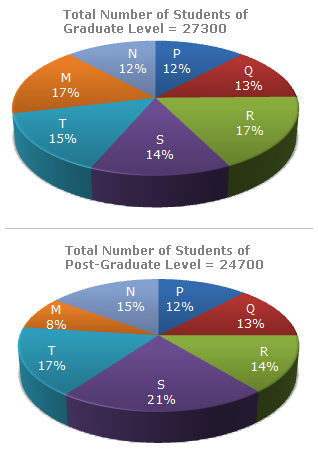# Data Interpretation - Pie Charts - Discussion

The following pie-charts show the distribution of students of graduate and post-graduate levels in seven different institutes in a town.1.

What is the total number of graduate and post-graduate level students is institute R?

 [A]. 8320 [B]. 7916 [C]. 9116 [D]. 8099

Explanation:

 Required number = (17% of 27300) + (14% of 24700) = 4641 + 3458 = 8099.

 Hrushikesh said: (Aug 15, 2010) its 17% of 27300 not 18% answer is therefore wrong..answer is 8099

 Swetha said: (Dec 14, 2013) I want the methods of solving the problems why we have taken m s % s.

 Ankita said: (Sep 8, 2015) How we will solve if the values 27300 and 24700 is not given?